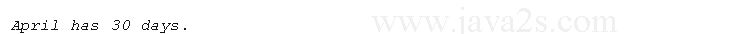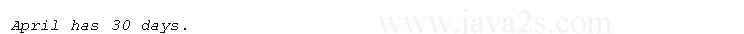# Java Array Type

## Introduction

An array is a list of same-typed variables.

Java arrays can be of any type and may have one or more dimensions.

A specific element in an array is accessed by its index.

## One-Dimensional Arrays

To create an array, create an array type variable.

The general form of a one-dimensional array declaration is

```type var-name[];
type[] var-name;
```

Note that we can place `[]` after `type` or after `var-name`.

After `type` is preferred since we know right way that the `type` is for array type.

For example, the following two declarations are equivalent:

```int al[] = new int;
int[] a2 = new int;

int[] nums, nums2, nums3; // create three arrays
int nums[], nums2[], nums3[]; // create three arrays
```

The following declarations are also equivalent:

```char twod1[][] = new char;
char[][] twod2 = new char;
```

Here, `type` declares the element type of the array.

For example, the following declares an array named `month_days` with the type "array of int":

```int[] month_days;
```

To create physical array of integers, we need to use `new` and assign it to `month_days`.

`new` is an operator that allocates memory.

The general form of new as it applies to one-dimensional arrays appears as follows:

```array-var = new type[size];
```

Here, `type` specifies the type of data being allocated.

`size` specifies the number of elements in the array.

`array-var` is the array variable name.

The elements in the array allocated by new will automatically be initialized to

• zero for numeric types
• false for boolean
• null for reference types

The following line of code allocates a 12-element array of integers and links them to month_days:

```month_days = new int;
```

After this statement executes, month_days will refer to an array of 12 integers.

All elements in the array will be initialized to zero.

Once you have allocated an array, you can access a specific element in the array by specifying its index.

All array indexes start at zero.

For example, this statement assigns the value 28 to the second element of month_days:

```month_days = 28;
```

The next line displays the value stored at index 3:

```System.out.println(month_days);
```
```// Demonstrate a one-dimensional array.
public class Main {
public static void main(String args[]) {
int month_days[];
month_days = new int;
month_days = 31;//from   w  ww  .  j  ava 2  s.  com
month_days = 28;
month_days = 31;
month_days = 30;
month_days = 31;
month_days = 30;
month_days = 31;
month_days = 31;
month_days = 30;
month_days = 31;
month_days = 30;
month_days = 31;
System.out.println("April has " + month_days + " days.");
}
}
```To combine the declaration of the array variable with the allocation of the array itself, as shown here:

```int month_days[] = new int;
```

Arrays can be initialized when they are declared.

An array initializer is a list of comma-separated expressions surrounded by curly braces.

The array will be created large enough to hold the number of elements in the array initializer.

There is no need to use new.

For example, to store the number of days in each month, the following code creates an initialized array of integers:

```public class Main {
public static void main(String args[]) {
int month_days[] = { 31, 28, 31, 30, 31, 30, 31, 31, 30, 31, 30, 31 };
System.out.println("April has " + month_days + " days.");
}/*w  ww.  j a va2s.c om*/
}
```